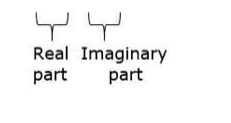# Simplify each of the following and express it in the form a + ib

Question:

Simplify each of the following and express it in the form a + ib :

$(8-4 i)-(-3+5 i)$

Solution:

Given: $(8-4 i)-(-3+5 i)$

Firstly, we open the brackets

$8-4 i+3-5 i$

$=11-9 i$